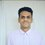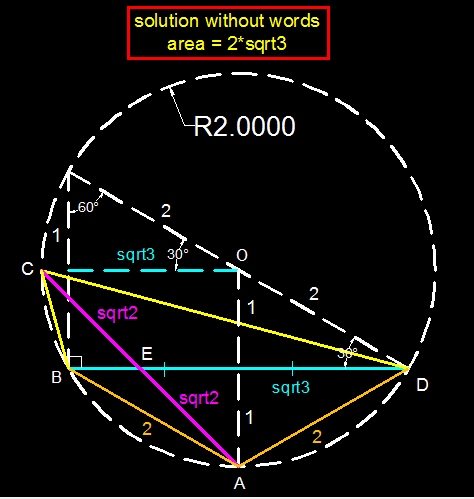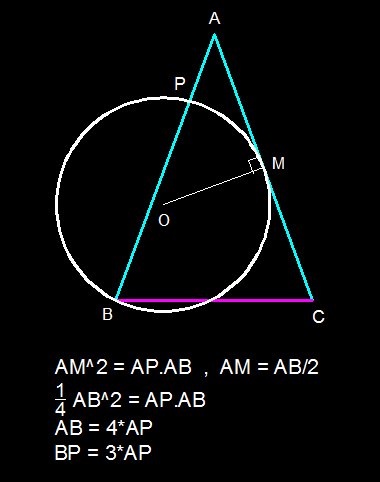# Xuming's Geometry Synthetic Group - Shivam's proposal

1. The circumcircle of the quadrilateral $ABCD$ has a radius 2. $AC, BD$ cut at $E$ such that $AE=EC$. If $AB=\sqrt{2} AE, BD=2\sqrt {3}$ . Find the area of quadrilateral $ABCD$.
2. Triangle ABC is an isosceles triangle in which AB=A C. A circle is drawn passing through B and touching AC at its midpoint M. The circle cuts AB at P . Prove that BP=3AP.
3. In an isosceles triangle the altitude drawn to the base is $\frac{2}{3}$ times the radius of the circumcircle . Prove the base angle of the triangle is $\text{arccos}\sqrt{\frac{2}{3}}$.
4. The sides of a right angled triangle are all integers . Two sides are prime that differ by 50.Find the smallest value of the third side.
5. In triangle ABC , D is on BC such that BD=3DC. E is on AC such that 3AE=2EC. AD and BE cut F. Area of triangle AFE=4 and area of triangle BFD=30 . Find area of triangle ABC.
6. In a circle AB is a diameter . AB is produced to P such that BP=Radius of the circle . PC is tangent to the circle . The tangent at B amd AC produced cut at E . Then describe triangle CDE.5 years, 10 months ago

This discussion board is a place to discuss our Daily Challenges and the math and science related to those challenges. Explanations are more than just a solution — they should explain the steps and thinking strategies that you used to obtain the solution. Comments should further the discussion of math and science.

When posting on Brilliant:

• Use the emojis to react to an explanation, whether you're congratulating a job well done , or just really confused .
• Ask specific questions about the challenge or the steps in somebody's explanation. Well-posed questions can add a lot to the discussion, but posting "I don't understand!" doesn't help anyone.
• Try to contribute something new to the discussion, whether it is an extension, generalization or other idea related to the challenge.

MarkdownAppears as
*italics* or _italics_ italics
**bold** or __bold__ bold
- bulleted- list
• bulleted
• list
1. numbered2. list
1. numbered
2. list
Note: you must add a full line of space before and after lists for them to show up correctly
paragraph 1paragraph 2

paragraph 1

paragraph 2

[example link](https://brilliant.org)example link
> This is a quote
This is a quote
    # I indented these lines
# 4 spaces, and now they show
# up as a code block.

print "hello world"
# I indented these lines
# 4 spaces, and now they show
# up as a code block.

print "hello world"
MathAppears as
Remember to wrap math in $$ ... $$ or $ ... $ to ensure proper formatting.
2 \times 3 $2 \times 3$
2^{34} $2^{34}$
a_{i-1} $a_{i-1}$
\frac{2}{3} $\frac{2}{3}$
\sqrt{2} $\sqrt{2}$
\sum_{i=1}^3 $\sum_{i=1}^3$
\sin \theta $\sin \theta$
\boxed{123} $\boxed{123}$

Sort by:

Q3:

Let the isosceles $\triangle ABC$ has $AB=AC$, its base angle be $\theta$ and the center and radius of the circumcircle be $O$ and $r$ respectively.

Then the extension of altitude $AP$ to $BC$ passes through $O$; $AP = \frac{2}{3}r$, $OP = \frac{1}{3}r$ and then $BP = \sqrt{OB^2-OP^2} = \sqrt{r^2 - \left(\frac{1}{3}r\right)^2} = \frac{2 \sqrt{2}}{3}$.

Therefore, $\tan{\theta} = \dfrac{AP}{BP} = \dfrac{\frac{2}{3}r}{\frac{2\sqrt{2}}{3}r} = \dfrac{1}{\sqrt{2}} \quad \Rightarrow \cos \theta = \sqrt{\frac {2}{3}} \quad \Rightarrow \theta = \boxed{\cos^{-1} \left( \sqrt{\frac {2}{3}}\right)}$

- 5 years, 10 months ago

Q5:

We note that $\triangle CFE$ has the same altitude as $\triangle AFE$, therefore their areas are in the ratio of the base lengths. That is:

$\dfrac{[\triangle CFE]}{[\triangle AFE]} = \dfrac{EC}{AE} = \dfrac{3}{2} \quad \Rightarrow [\triangle CFE] = \dfrac{3}{2} \times [\triangle AFE] = \dfrac{3}{2} \times 4 = 6$

Similarly, $[\triangle CFD] = \dfrac{DC}{BD} \times [\triangle BFD] = \dfrac{1}{3} \times 30 = 10$

And, $[\triangle ABD] = \dfrac{BD}{DC} \times [\triangle ACD] = 3 \times ([\triangle AFE]+[\triangle CFE]+[\triangle CFD]) = 3 \times (4+6+10) = 60$

Therefore, $[\triangle ABC] = [\triangle ABD] + [\triangle ACD] = 60 + 20 = \boxed{80}$

- 5 years, 10 months ago

Q:1Q:2- 4 years, 2 months ago